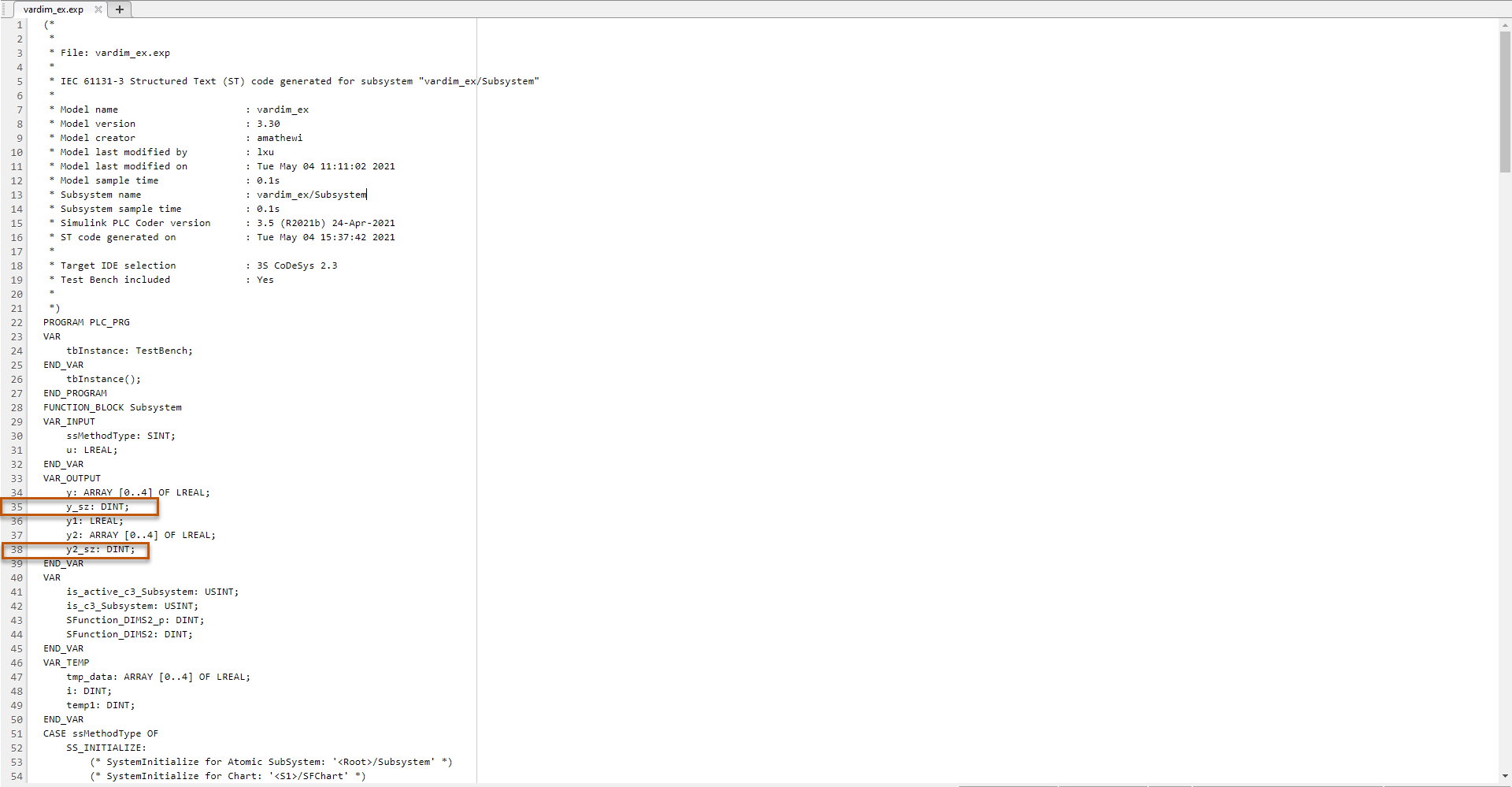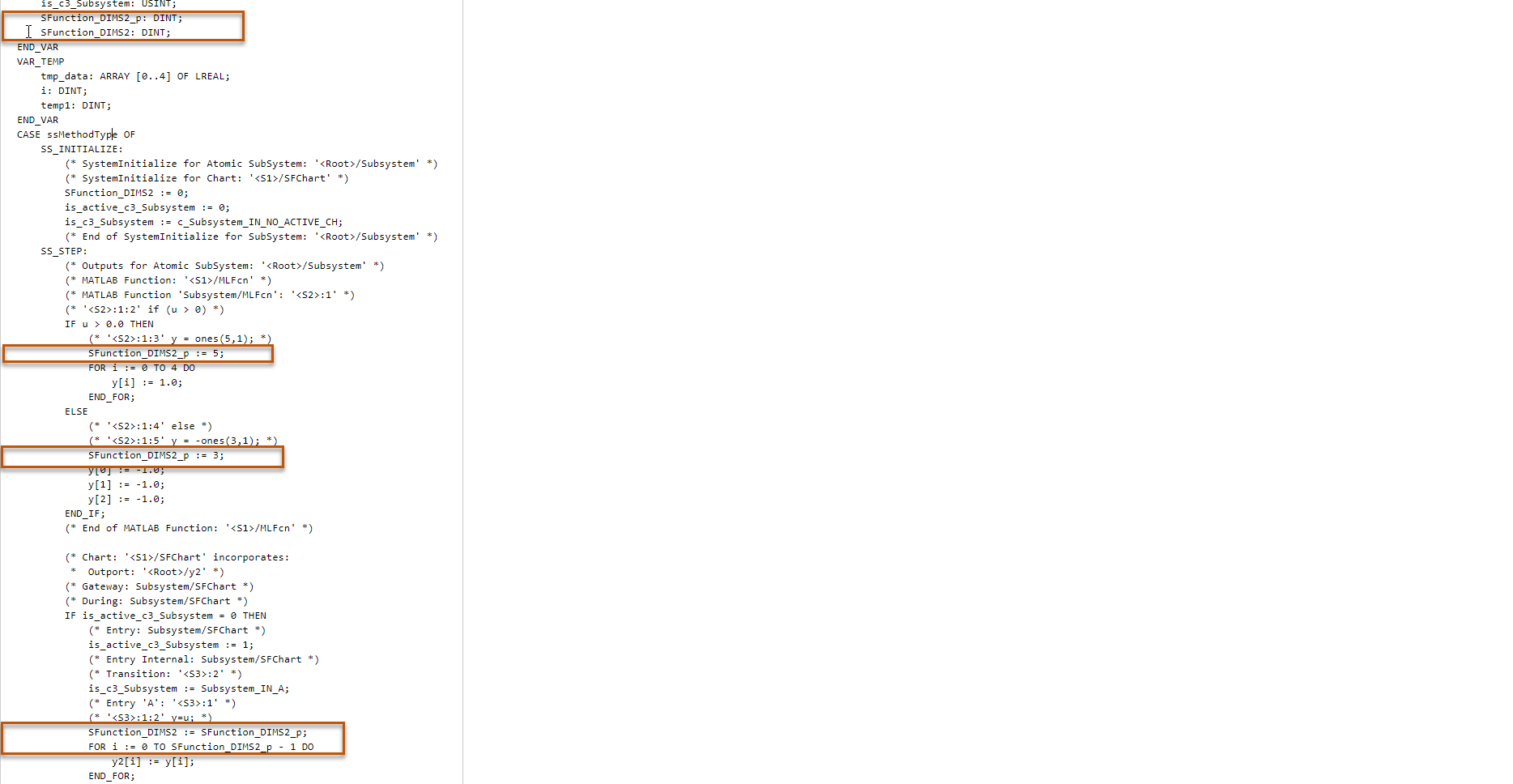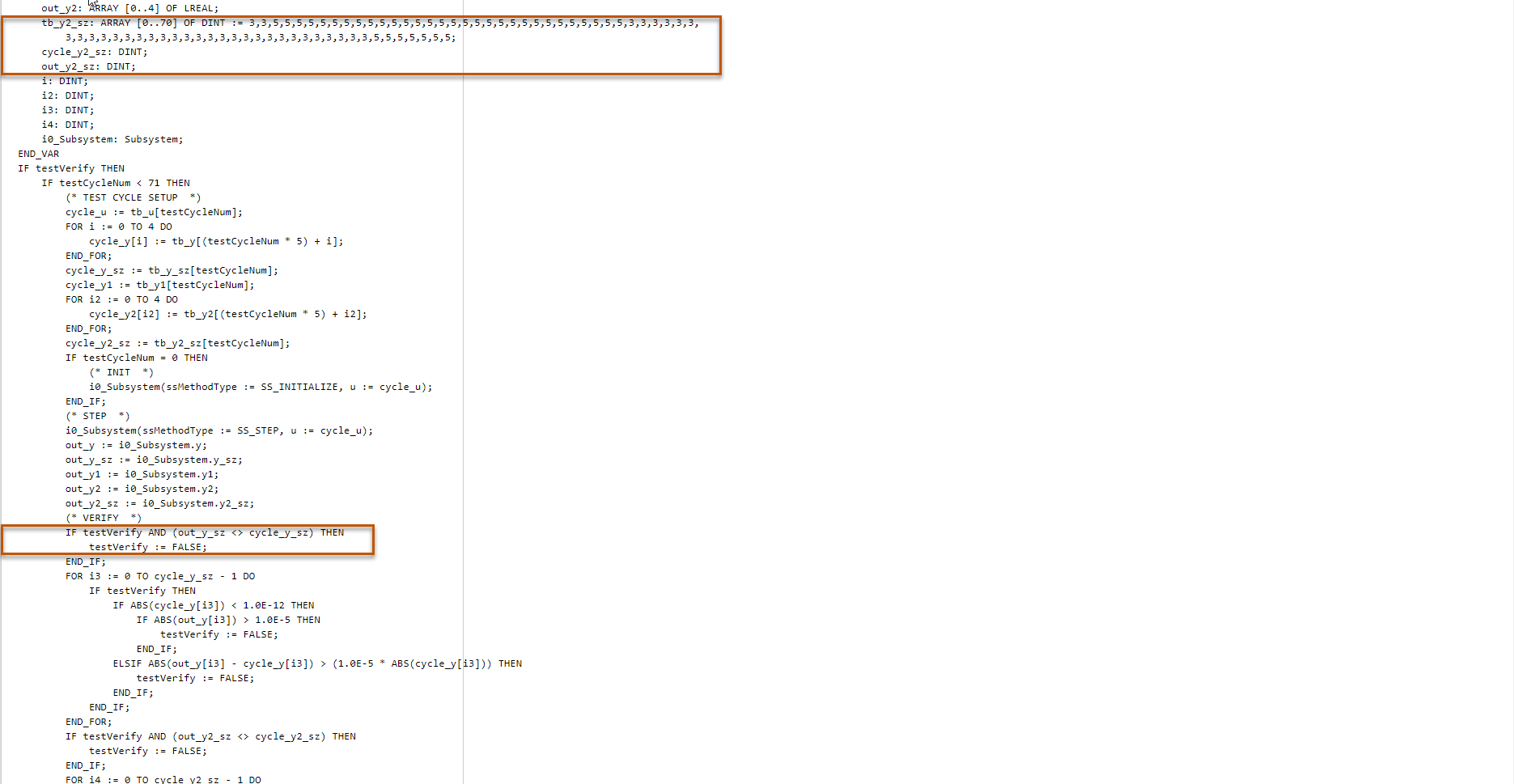## Variable-Size Signal Code Generation

To generate code for your Simulink® subsystems, Stateflow® charts, and MATLAB® Function Blocks that have variable-size signals, use Simulink PLC Coder™. An array that has at least one variable dimension is called a variable-size array. Variable-size arrays are commonly found in applications such as machine learning and deep learning. Construct variable-size signals in your model by using Simulink blocks, MATLAB Function Blocks, and Stateflow charts. For more information, see Variable-Size Signal Basics.

### Limitations

Simulink PLC Coder does not support variable-size code generation for:

• Subsystems that do not have the Function Packaging set to `Inline`.

• Do not use one-dimensional variable-size inputs and outputs in the top-level subsystem.

• Selector blocks that have variable-size data.

Simulink PLC Coder supports only these model components for variable-size code generation:

• MATLAB Function Blocks

• Stateflow charts

### Variable-Size Code Generation Example

To generate code for a model with variable-size signals, see Generate Structured Text Code for Variable-Size Signals.

### Generated Code Structure for Variable-Size Signals

For the example Generate Structured Text Code for Variable-Size Signals, these images show the structure of the code generated for the model.

This image shows the highlighted output variables for the variable-size data MATLAB Function Block.This image shows the highlighted output variables for the Stateflow chart.This image shows the highlighted generated variable-size code for the generated test bench.